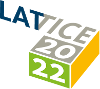#The 39th International Symposium on Lattice Field Theory (Lattice 2022)

Aug 8 – 13, 2022
Hörsaalzentrum Poppelsdorf
Europe/Berlin timezone

## Electromagnetic form factors of the proton and neutron from $N_\mathrm{f} = 2 + 1$ lattice QCD

Aug 9, 2022, 2:00 PM
20m
CP1-HSZ/1.002 (CP1-HSZ) - HS6 (CP1-HSZ)

50
Show room on map

### Speaker

Mr Miguel Salg (University of Mainz)

### Description

We present results for the electromagnetic form factors of the proton and neutron computed on the Coordinated Lattice Simulations (CLS) ensembles with $N_\mathrm{f} = 2 + 1$ flavors of $\mathcal{O}(a)$-improved Wilson fermions and an $\mathcal{O}(a)$-improved conserved vector current. In order to estimate the excited-state contamination, we employ several source-sink separations and apply the summation method. The quark-disconnected diagrams entering the isoscalar quantities are computed explicitly. For this purpose, a stochastic estimation based on the one-end trick is performed, in combination with a frequency-splitting technique and the hopping parameter expansion. By these means, we obtain a clear signal for the form factors including the quark-disconnected contributions, which have a statistically significant effect on our results. From the $Q^2$-dependence of the form factors, we determine the electric and magnetic charge radii and the magnetic moment of the proton and neutron. The chiral interpolation is carried out by simultaneously fitting the pion mass and $Q^2$-dependence of our form factor data directly to the expressions resulting from covariant chiral perturbation theory including vector mesons. To assess the influence of systematic effects, we average over various cuts in the pion mass and the momentum transfer, as well as over different models for the lattice spacing dependence, using weights derived from the Akaike Information Criterion (AIC).

### Primary author

Mr Miguel Salg (University of Mainz)

### Co-authors

Dalibor Djukanovic Georg von Hippel (JGU Mainz) Harvey B. Meyer (Johannes Gutenberg University Mainz) Konstantin Ottnad (University of Mainz) Hartmut Wittig